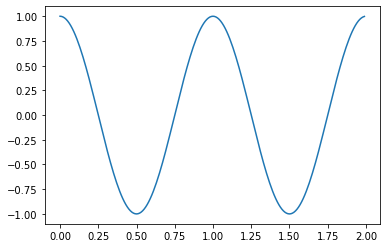Question #659

# What is the simplest example with Matplotlib?

Merged questions
Answer

The following Python code is probably the simplest plot with Matplotlib:

``````import matplotlib.pyplot as plt
import numpy as np

# Prepare data y=cos(2π.x)
x = np.arange(0.0, 2.0, 0.01)
y = np.cos(2 * np.pi * x)

# Create figure and plot data
fig, ax = plt.subplots()
ax.plot(x, y)``````

The above code should display the following chart:Note that you can plot directly with ﻿`plt.plot(x,y)`, but it's a good habit to collect ﻿`fig` and ﻿`ax` for future use (displaying grid, axis label, title ...).

2 events in history
Answer by Alphonsio 02/08/2021 at 06:14:52 PM

The following Python code is probably the simplest plot with Matplotlib:

``````import matplotlib.pyplot as plt
import numpy as np

# Prepare data y=cos(2π.x)
x = np.arange(0.0, 2.0, 0.01)
y = np.cos(2 * np.pi * x)

# Create figure and plot data
fig, ax = plt.subplots()
ax.plot(x, y)``````

The above code should display the following chart:Note that you can plot directly with ﻿`plt.plot(x,y)`, but it's a good habit to collect ﻿`fig` and ﻿`ax` for future use (displaying grid, axis label, title ...).

Question by Alphonsio 02/08/2021 at 06:09:06 PM
What is the simplest example with Matplotlib?

Icons proudly provided by Friconix.